Courses

# Differential Equations Civil Engineering (CE) Notes | EduRev

## Civil Engineering (CE) : Differential Equations Civil Engineering (CE) Notes | EduRev

The document Differential Equations Civil Engineering (CE) Notes | EduRev is a part of the Civil Engineering (CE) Course Topic wise GATE Past Year Papers for Civil Engineering.
All you need of Civil Engineering (CE) at this link: Civil Engineering (CE)

Q.1 An ordinary differential equation is given below.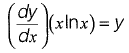The solution for the above equation is
(Note: K denotes a constant in the options)
(a) y = Kxex
(b) y = Kxe-x
(c) y = Klnx
(d) y = Kxlnx     [2019 : 2 Marks, Set-II]
Ans:
(c)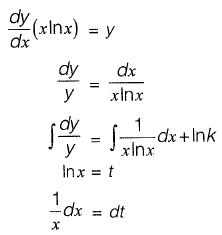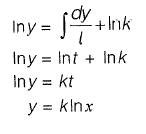Q. 2 Consider the ordinary differential equation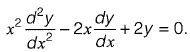Given the values of y(1) = 0 and y(2) = 2, the value of y{3) (round off to 1 decimal place), is_______     [2019 : 2 Marks, Set-I]
Ans: y{3} = (6)

Q. 3 A one-dimensionai domain is discretized into N sub-domains of width Δx with node numbers i = 0,1,2, 3 ....... N. If the time scale is discretized in steps of Δt, the forward-time and centered- space finite difference approximation at nth node and time step, for the partial differential equation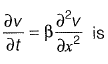(a)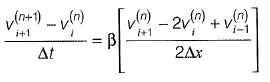(b)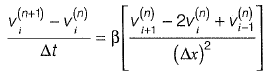(c)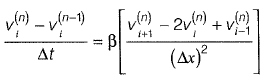(d)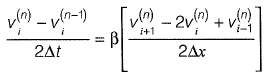[2019 : 2 Marks, Set-I]

Ans: (b)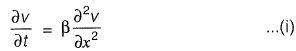Approximate time derivative in equation (i) with forward difference,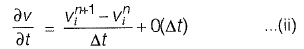Note that the right hand side only in value v at x = xi
Use the central difference approximation to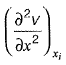and evaluate all the terms at time n.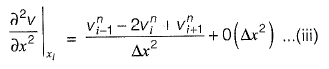Substituting equation (ii) in the left hand side of equation (i), substitute the equation (iii) into the right hand side of equation (i), and collect the truncation error terms to get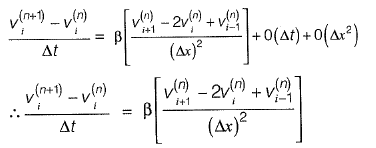Q. 4 The solution of the equation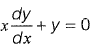passing through the point (1,1) is

(a) x
(b) x2
(c) x-
(d) x-2     [2018 : 1 Mark, Set-II]

Ans: (c)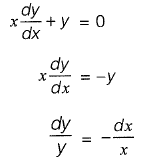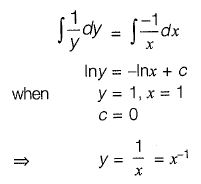Q. 5 The solution (up to three decimal places) at x = 1 of the differential equation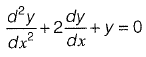subject to boundary conditions y(0) = 1 and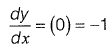[2018 : 2 Marks, Set-I]
Ans: 0.368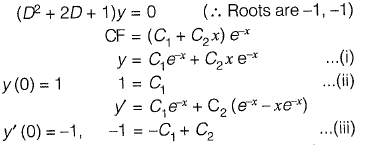From eq. (ii) and (iii),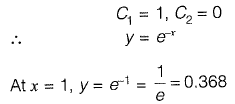Q. 6 Consider the following second-order differential equation:
y "- 4y' + 3 y = 2t - 3t2

The particular solution of the differential equation is
(a) - 2 - 2t - t2
(b) - 2t - t2
(c) 2t - t2
(d) - 2 - 2t - 3t2       [2017 : 2 Marks, Set-II]
Ans: (a)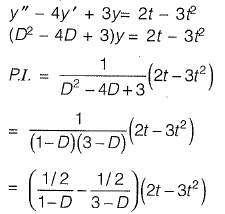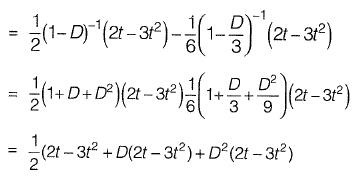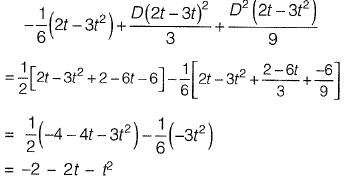Q. 7 The solution of the equation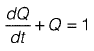with Q = 0 at t = 0 is
(a) Q(t) = e-t - 1
(b) Q(t) = 1 + e-t
(c) Q(t) = 1 - et

(d) Q(f) = 1 - e-t        [2017 : 2 Marks, Set-I]
Ans : (d)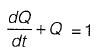comparing with standard form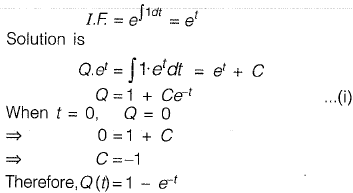Q. 8 Consider the following partial differential equation: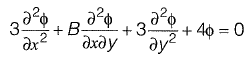For this equation to be classified as parabolic, the value of B2 must be ________ .       [2017 : 1 Mark, Set-I]
Ans: 36

Given that the partial differential equation is parabolic.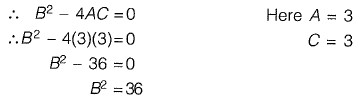Q. 9 The respective expressions for complimentary function and particular integral part of the solution of the differential equation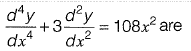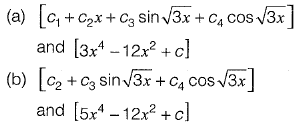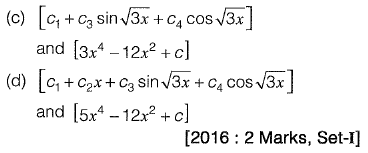Ans: a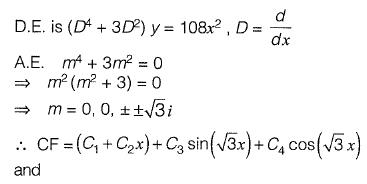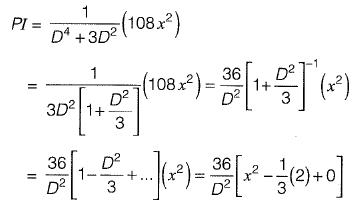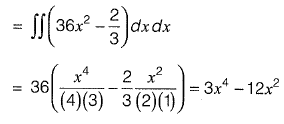Q. 10 The solution of the partial differential equation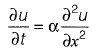is of the form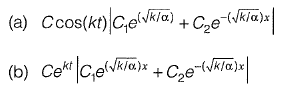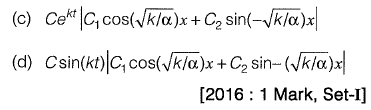Ans: (b)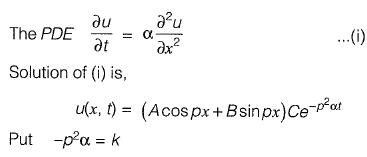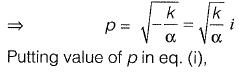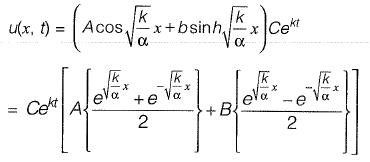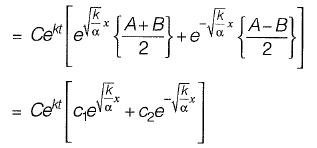Q. 11 The type of partial differential equation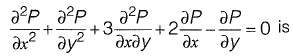(a) elliptic
(b) parabolic
(c) hyperbolic
(d) none of these    [2016 : 1 Mark, Set-I]
Ans: (c)
Comparing the given equation with the general form of second order partial differential equation, we have A = t , B = 3, C = 1
=> B2 - 4AC = 5 > 0
∴ PDE is Hyperbola.

Q. 12 Consider the following second order linear differential equation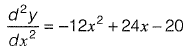The boundary conditions are: at x = 0, y = 5 and x = 2, y = 21
The value of y at x = 1 is .    [2015 : 2 Marks, Set-II]

Ans: y = 18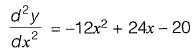Integrating both sides wrt. x,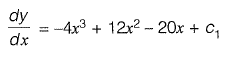Integrating both sides wrt. x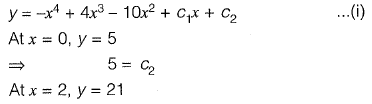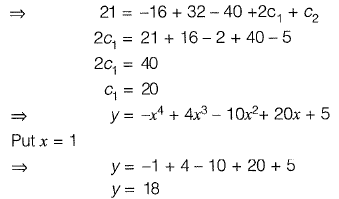Q. 13 Consider the following difference equation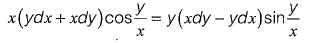Which of the following is the solution of the above equation (c is an arbitrary constant)?          [2015 : 2 Marks, Set-I]
(a)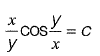(b)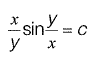(c)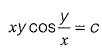(d)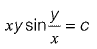Ans: c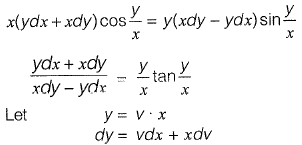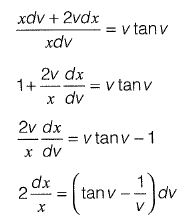Integrating both side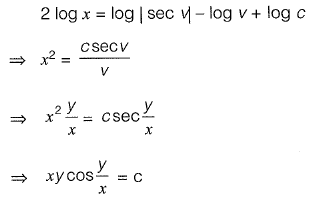Q. 14 The integrating factor for differential equation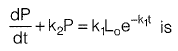[2014 : 1 Mark, Set-II]
(a)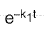(b)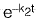(c)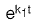(d)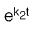Ans: (d)

Q. 15 The solution of the ordinary differential equation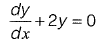for the boundary condition, y = 5 at x = 1 is     [2011 : 2 Marks]
(a) y = e-2x
(b) y = 2e-2x
(c) y = 10.95 e-2x
(d) y = 36.95 e-2x

Ans: (d)

Given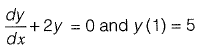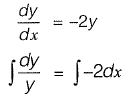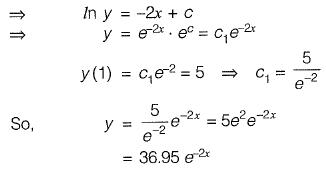Q. 16 The solution of the diffrential equation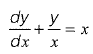with the condition that y = 1 at x = 1 is    [2011 : 2 Marks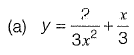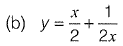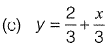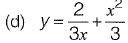Ans: (d)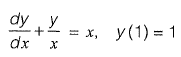This is a linear differential equation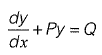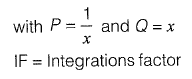solution is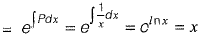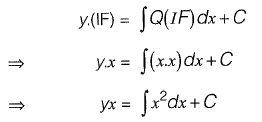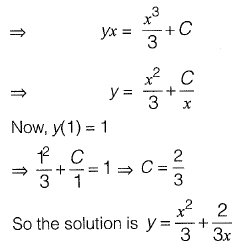Q. 17 A parabolic cable is held between two supports at the same level. The horizontal span between the supports is L. The sag at the mid-span is h. The equation of the parabola is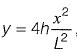where x is the horizontal coordinate and y is the vertical coordinate with the origin at the centre of the cable. The expression for the total length of the cable is
[2010 : 2 Marks]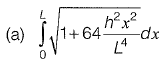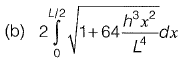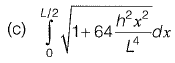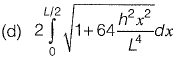Ans: (d)

Length of curve f(x) between x = a and x = b is given by,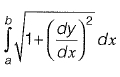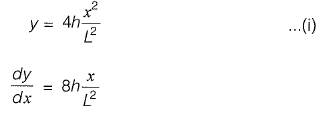since, y = 0 at x= 0
and y = h at x = L/2
(As can be seen from equation (i), by substituting x = 0 and x = L/2)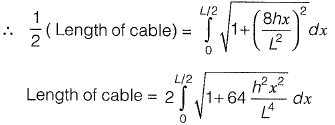Q. 18 The partial differential equation that can be formed from z = ax + by + ab has the form (with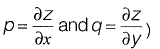[2010 : 2 Marks]

(a) z = px + qy
(b) z = px + pq
(c) z = px + qy + pq
(d) z = qy + pq

Ans: (c)

z = a x + b y + a b ...(i)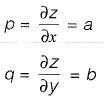Substituting a and b in (i) in terms of p and q, we get,
z = px + qy + pq

Q. 19 The solution to the ordinary differential equation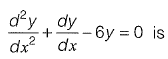[2010 : 2 Marks]
(a) y= c1e3x + c2e-2x
(b) y= c1e3x + c2e2x
(c) y= c1e-3x + c2e2x
(d) y= c1e-3x + c2e-2x
Ans: (c)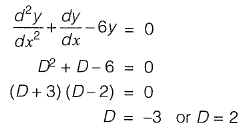∴ Solution is y = = c1e-3x + c2e2x

Q. 20 The order and degree of the differential equation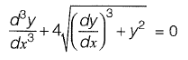are respectively    [2010 : 1 Mark]
(a) 3 and 2
(b) 2 and 3
(c) 3 and 3
(d) 3 and 1
Ans (a)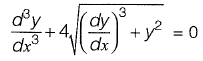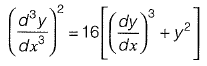∴ The order is 3 since highest differential is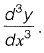The degree is 2 since power of highest differential is 2.

Offer running on EduRev: Apply code STAYHOME200 to get INR 200 off on our premium plan EduRev Infinity!

67 docs

,

,

,

,

,

,

,

,

,

,

,

,

,

,

,

,

,

,

,

,

,

;